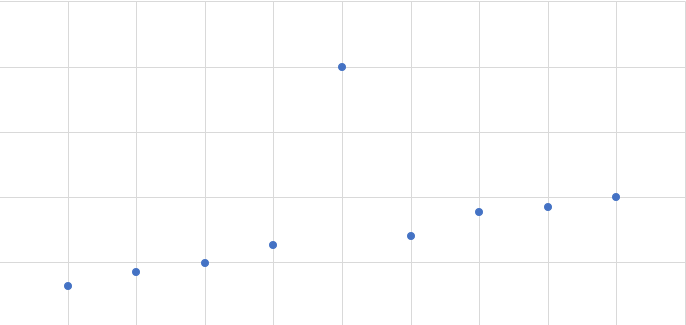# 线性回归(二)-违背基本假设的情况和处理方法

## 基本假设

1. 样本的显著性检验：若已知总体方差则可使用t检验等参数检验；若总体方差未知，则可以使用卡方检验等非参数检验
2. 回归方程的检验：回归的显著性检验，对于回归参数采用t分布进行检验；对于回归方程采用F检验

## 违背基本假设的处理

### 随机误差项方差为异方差

#### 异方差的检验做$y$关于$x$的线性回归，计算$\varepsilon$的估计值${e_i} = {y_i} - {{\hat y}_i}$

将自变量$x_i$与$e_i$按升序或降序的规则排列，按照排列顺序相同等级的自变量和残差计算等级离差${d_i} = {x_i} - |{e_i}|$

计算等级相关系数${r_s} = 1 - \frac{6}{{n*({n^2} - 1)}}\sum {d_i^2}$，由方程可见，当残差和自变量等级变化趋势完全拟合时，相关系数等于1。若$d_i$差异较大时，则$r_s$越小。查阅资料得，等级相关系数的期望等于0，方程为$1-{r_s}^2$。

根据其期望和方差构造$t$统计量，$t = \frac{{\sqrt {n - 2} }}{{\sqrt {1 - r_S^2} }}{r_S}$。可以选择相应的置信区间进行单侧检验。若检验通过则认为随机误差项的方差等于常数，否则存在异方差的问题。

#### 异方差的消除

$$\begin{array}{l} {x_{\omega i}} = {\omega _i}{x_i}\\ {y_{\omega i}} = {y_i}\\ {{\bar x}_\omega } = \frac{{\sum {{\omega _i}{x_i}} }}{{\sum {{\omega _i}} }}\\ {{\bar y}_\omega } = \frac{{\sum {{\omega _i}{y_i}} }}{{\sum {{\omega _i}} }} \end{array}$$

$$\left\{ {\begin{array}{*{20}{c}} {{{\hat \beta }_{\omega 0}} = {{\bar y}_\omega } - {{\hat \beta }_{w1}}{{\bar x}_\omega }}\\ {{{\hat \beta }_{w1}} = \frac{{\sum {{w_i}({x_i} - {{\bar x}_\omega })({y_i} - {{\bar y}_\omega })} }}{{\sum {{w_i}{{({x_i} - {{\bar x}_\omega })}^2}} }}} \end{array}} \right.$$

### 自相关的检验和处理

#### 自相关的检验方法

$$\rho = \frac{{\sum {{\varepsilon _t}{\varepsilon _{t - 1}}} }}{{\sqrt {\sum {{\varepsilon _t}^2} \sum {\varepsilon _{t - 1}^2} } }}$$ 其中$t \ge 2$。

DW检验法（重点）

DW检验法适用于小样本的一阶的自回归检验方法。

$$DW = \frac{{\sum {{{({e_t} - {e_{t - 1}})}^2}} }}{{\sum {e_t^2} }} \approx 2(1 - \hat \rho )$$

$\rho$ DW 自相关性
-1 4 完全负相关
(-1, 0) (2, 4) 负自相关
0 2 无自相关
(0, 1) (0, 2) 正相关
1 0 完全正相关

#### 消除自相关的方法

$$\begin{array}{l} ({y_t} - \hat \rho *{y_{t - 1}}) = ({\beta _0} - \hat \rho {\beta _0}) + {\beta _1}({x_t} - \hat \rho {x_{t - 1}}) + ({\varepsilon _t} - \hat \rho {\varepsilon _{t - 1}})\\ = > {y_t}' = {\beta _0}' + {\beta _1}'{x_t}' + {u_t} \end{array}$$

$$\begin{array}{l} 令：\Delta y = {y_t} - {y_{t - 1}}\\ \Delta x = {x_t} - {x_{t - 1}}\\ {\rm{ = > }}\Delta y{\rm{ = }}{\beta _{\rm{0}}}{\rm{ + }}{\beta _{\rm{1}}}\Delta x \end{array}$$ 参数的估计公式为：${{\hat \beta }_{\rm{0}}} = 0,{{\hat \beta }_1} = \frac{{\sum {\Delta {x_i}\Delta {y_i}} }}{{\sum {\Delta x_i^2} }}$

BOX-COX变换法：

将因变量做一定的变换：${y^{(\lambda )}} = \left\{ {\begin{array}{*{20}{c}} {\frac{{{y^\lambda } - 1}}{\lambda },\lambda \ne 0}\\ {\ln y,\lambda = 0} \end{array}} \right.$

应用极大似然估计法进行求解：

$$\begin{array}{l} {L_{\max }}(\lambda ) = {(2\pi e\hat \sigma _\lambda ^2)^{ - \frac{n}{2}}}|J|\\ 其中：\hat \sigma _\lambda ^2 = \frac{1}{n}SSE(\lambda ,{y^{(\lambda )}}),|J| = \prod {|\frac{{dy_i^{(\lambda )}}}{{d{y_i}}}|} = \prod {y_i^{\lambda - 1}} \\ 令：{z^{(\lambda )}} = \frac{{{y^{(\lambda )}}}}{{|J|}} \end{array}$$

### 异常值的检查与消除

#### 异常值得判定

##### 异常值的常见情况和消除方法对残差进行标准化，即$ZR{E_i} = \frac{{{e_i}}}{{\hat \sigma }}$。该标准化的目的是统一残差的偏离程度，即标准化后的样本方差等于1，减少异方差的影响

删除偏离较大的残差，若样本数量足够，可以在一定程度上通过删除该异常值来达到忽略异常情况对拟合质量的影响。

学生化残差，$SR{E_i} = \frac{{{e_i}}}{{\sigma \sqrt {1 - {h_{ii}}} }}$，其中$h_{ii}$为帽子矩阵主对角线上的元素，帽子矩阵计算：$H = X{({X^T}X)^{ - 1}}{X^T}$。其主对角线上的元素为变量Y与自变量X期望的偏离程度。

删除学生化残差：学生化残差之后，残差的分布即变成：$e\~N(0,1)$，其满足正态分布，按照正态分布的中心化原则，当学生化的残差$e_t$的绝对值大于3时，可以将该残差进行删除。

当帽子矩阵对应杠杆值的$h_{ii}$较大时，可认为当前自变量X取值与因变量取值受影响较大，当杠杆值大于2倍或3倍的平均值${\bar h}$时，可认为该值异常。

计算库克距离：${D_i} = \frac{{e_i^2}}{{(n + 1){{\hat \sigma }^2}}}*\frac{{{h_{ii}}}}{{{{{\rm{(1 - }}{h_{ii}}{\rm{)}}}^{\rm{2}}}}}$，库克距离反映的是残差和杠杆值的综合效应，当库克距离越小，表明值的偏差越小。因此取库克值小于0.5认为非异常值，值大于1认为为异常值，即${D_i}{\rm{ < 0}}{\rm{.5}}$非异常，${D_i}{\rm{ > 1}}$异常值。

## 多元线性回归下的自变量选择与处理

### 自变量选择方法和影响

1. 最后的计算得到的参数估计值的方差为有偏估计
2. 部分因素的选择模型方差会小于全因素模型的方差

### 自变量选择的评价指标

$$\begin{array}{l} {C_p} = \frac{{SS{E_p}}}{{{{\hat \sigma }^2}}} - n + 2p\\ = (n - m - 1)\frac{{SS{E_p}}}{{SS{E_m}}} - n + 2p \end{array}$$

### 自变量的选择方式

1. 先对所有的单变量因素进行拟合，然后根据拟合结果计算上述4个统计量，得到效果最好的一个变量；
2. 考虑上一步增加的变量，在剩余变量中选取一个与当前选取变量组合，计算所有的组合情况，并选出最优组合与先前组合进行了比较，若更优则选取，若更差则放弃；
3. 考虑上一步增加的变量，再次在剩余变量中选取并组合，计算组合情况选择最优，并与与上次组合结果比较优劣；
4. 循环第3步，直到遍历变量全部完成或当优化程度下降时停止，得到最优组合。

1. 先将所有的因素进行分析计算，保存四个统计量
2. 删除其中一个变量的影响因素，并对进行分析计算，找出删除一个自变量的最优组合，并于先前组合进行对比。若结果更好则继续，若更差则停止。
3. 循环第2步直到自变量数量为一，或删除变量结果变坏为止。

## 多重共线性的处理

### 多重共线性的检测和处理

#### 皮尔逊相关系数检验法

1. 添加或删除一个变量，回归系数的估计值发生很大变化，可认为存在多重共线性。因为多重共线性的参数会叠加到其他共线变量的参数上。
2. 一些自变量在方程的显著性检验中未通过检验，可初步判定存在严重的多重共线性。

#### 方差扩大因子法VIF

$${R_{p \times p}} = \left[ {\begin{array}{*{20}{c}} {r11}&{r12}&{...}&{r1p}\\ {r21}&{r22}&{...}&{r2p}\\ {...}&{...}&{...}&{...}\\ {rp1}&{rp2}&{...}&{rpp} \end{array}} \right]$$

$$\begin{array}{l} VI{F_j} = \frac{1}{{1 - {R_j}^2}}\\ \bar V\bar I\bar F = \frac{1}{p}VI{F_j} \end{array}$$

为矩阵上第i个主对角线上的元素对应得复决定系数

$VIF_j$为膨胀因子。$\bar V\bar I\bar F$为平均膨胀因子。当膨胀银子大于10或平均膨胀因子大于1时，可认为自变量之间存在严重的线性关系，即存在多重共线性。

## 多元线性回归的优化方法

### 岭回归1. 各回归系数的岭迹基本稳定
2. 回归系数的符号合理
3. 取岭迹的平稳拐点
4. 方差扩大因子法，使得所有的膨胀系数小于10
5. 通过残差平方和最小确定k值

### LASSO回归<span class=”hint—top hint—rounded” aria-label=”LASSO回归法

-“></span></a></sup>

LASSO是一种压缩估计，通过构造一个惩罚函数(L1范数)，得到一个较为精炼的模型。

LASSO回归的计算公式相当复杂，一般通过坐标下降法进行迭代求解，或使用最小角回归法。进行计算求解。

## 参考资料

https://www.eatrice.cn/post/违背基本假设的情况和处理方法/

2020年4月10日